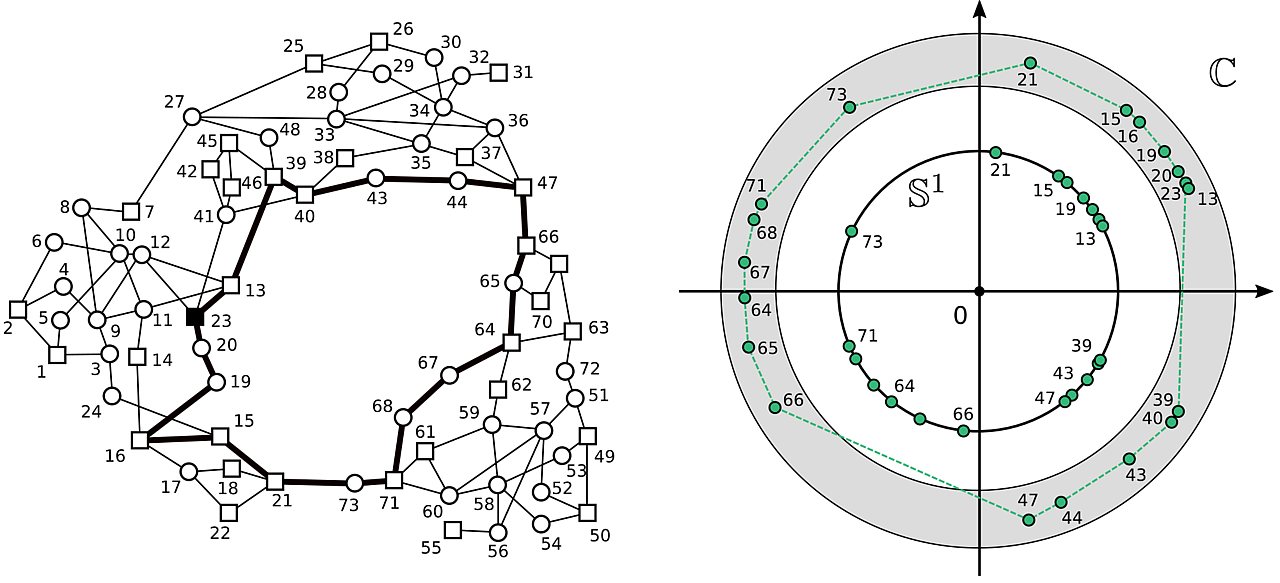# Multistability and anomalies in oscillator models of lossy power grids

The way power flows distribute over a complex power grid is puzzling to say the least. Over the last century, scientists have developed a variety of mathematical models, with various levels of refinement, attempting to describe and predict these flow distributions.In the electrical engineering community, the relation between voltage and power in an AC power grid is typically described by the power flow equations. Whereas these equations are rather easy to derive from fundamental laws of physics, solving them cannot be done in closed form. Engineers and operators who require to solve the power flow equations then rely on numerical schemes to approximate their solution. One drawback of such approaches is that it comes with very few guarantees about existence and uniqueness of the aforementioned solution.

Actually, it is known at least since the work of Korsak in 1972 , that the power flow equations can admit multiple solutions. Over that last decades, the relation between the number of solutions to the power flow equations and the underlying network structure of the grid have been gradually clarified. However, such clarifications came to a price, namely, the adoption of a series of simplifying assumptions, rendering the mathematical models less realistic.

One important simplification has been to neglect losses over high-voltage transmission lines. Under this lossless approximation, the solutions of the power flow equations are precisely the synchronous states of the celebrated Kuramoto model , describing the synchronization of a network of coupled phase oscillators. This framework allowed the physics and engineering communities to identify a clear relation between the graph describing the interactions between oscillators (i.e., the power grid) and the number and characteristics of power flow solutions [3-5].

Nowadays, the objective of the community is to figure out to what extent the results obtained under the lossless approximation extend to more realistic version of the power flow equations. In our Nature Communications paper "Multistability and anomalies in oscillator models of lossy power grids", we show that most of the lessons learned for lossless systems extend naturally to lossy systems. Whereas the final extension is intuitive and natural, the rigorous proof of its validity required a lot of technical machinery that we present in the paper.

On top of these fundamental results, we highlight a series of unexpected behaviors of the solutions to the power flow equations that are induced by the introduction of losses in the system. Namely, we show that more dissipation in a system does not necessarily jeopardize stability and transmission capacity.

 A. J. Korsak, "On the question of uniqueness of stable load-flow solutions," IEEE Trans. Power App. Syst. 91, 1093-1100 (1972).
 Y. Kuramoto, "Cooperative dynamics of oscillator community a study based on lattice of rings," Prog. Theor. Phys. Suppl. 79, 223-240 (1984).
 D. Manik, M. Timme, and D. Witthaut, "Cycle flows and multistability in oscillatory networks," Chaos 27, 083123 (2017).
 R. Delabays, T. Coletta, and P. Jacquod, "Multistability of phase-locking and topological winding numbers in locally coupled Kuramoto models on single-loop networks," J. Math. Phys. 57, 032701 (2016).
 S. Jafarpour, E. Y. Huang, K. D. Smith, and F. Bullo, "Flow and elastic networks on the n-torus: Geometry, analysis, and computation," SIAM Review 64, 59-104 (2022).

The paper is now published as:

R. Delabays, S. Jafarpour, and F. Bullo, "Multistability and anomalies in oscillator models of lossy power grids", Nat. Commun. 13, 5238 (2022). DOI: 10.1038/s41467-022-32931-8